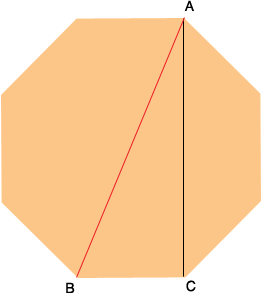SEARCH HOMEMath Central Quandaries & QueriesQuestion from cody: I have an octagon 3ft tall by 3ft wide. What are the lengths of the diagonal lines to the vertical and horizontal lines?Hi Cody,

I'm going to assume you mean a regular octagon. Are you looking for the length of AB, the red line in the diagram?If so, then since ABC is a right triangle you can use Pythagoras theorem. You know the length of CA is 3 feet so all that remains is to find the length of BC.

Look at the first diagram in my response to a previous question. The length of BC is W - 2b in that diagram.

Can you complete the problem from here?

PennyMath Central is supported by the University of Regina and The Pacific Institute for the Mathematical Sciences.